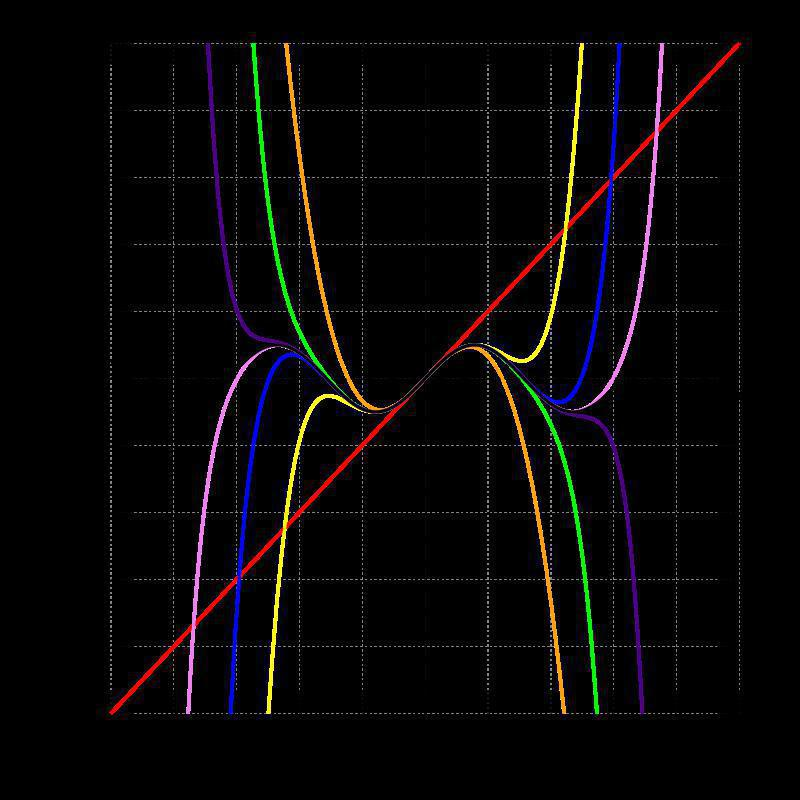# Taylor Expansions in PDEsEver wondered what the uses for taylor expansions are in the field of differential equations? no? well you should, its rather facinating…

First, what is a taylor expansion? well, basically, it says if youre trying to evaluate a function at a point that’s “close enough” to a point you already know you’ll be able to represent this slight difference as an infinate series:

$(1)\quad f(x+h)=f(x)+hf'(x)+\frac{h^2}{2!}f''(x)+O(h^3)$ Or if youre more comfortable with summation notation: $(2)\quad f(x+h)=\sum^{\infty}\_{n=0}\frac{(h)^n}{n!}f^{(n)}(x)$

Here $$O(h^3)$$ represents an arbitary function of order $$h^3$$ (basically just a function in $$h$$ with the smallest $$h$$ term being $$h^3$$), this is important and we will use this in a bit…

So how does this relate to a PDE? Well, we can estemate derivatives of a functon using this method, right? (as long as everything is nicly behaved) take eqn (1) above, and rearange it,get fid of a few terms & this will give you:

$(3)\quad f'(x)=\frac{f(x+h)-f(x)}{h}-\frac{h}{2!}f''(x)+O(h^2)$ or setting $$F’(x)\approx f’(x)$$ $(4)\quad F'(x)=\frac{f(x+h)-f(x)}{h}$

From here we can see that we can somewhat accuratly calculate a derivative of a function using the evaluation at 2 points that are “close enough” together (this can be done in both directions by using $$-h$$ insted). That’s cool, but now what?See this grid? This is a plane where a PDE lives. We can solve the PDE at a point on this plane if we use the above fomulas in a clever way. Imagine were ust working on the line $$y=0$$ and we want to work out $$f’(0.3)$$ where $$f(x)=x^2$$, how do we do this?

1. Remember that we can aprox $$f’(x)$$ using (4).
2. Set $$h$$ to be 0.1, or the spacing between the grid points.
3. Then we get $F'(0.3)=\frac{f(0.3+0.1)-f(0.3)}{0.1}$
4. Which we get $$f’(0.3)\approx F’(0.3)=\frac{(0.4)^2-(0.3)^2}{0.1}= 0.7 \approx 2(0.3)$$
5. And we have our approxamate answer at $$x=0.3$$

We can even make this more accurate by taking our expansion in $$+h$$ and finding the difference from our expansion in $$-h$$. essentially taking the difference between the 2 points either side of the position, $$x$$, we’re looking at. we get:

Forward: $$f(x+h)=f(x)+hf’(x)+\frac{h^2}{2!}f’‘(x)+O(h^3)$$
Backward: $$f(x-h)=f(x)-hf’(x)+\frac{(-h)^2}{2!}f’‘(x)+O(h^3)$$

so we can take the difference: $f(x+h)-f(x-h)=+2hf'(x)+O(h^3)$ $\Rightarrow f'(x)=\frac{f(x+h)-f(x-h)}{2h}+O(h^2)$ or setting $$F’(x)\approx f’(x)$$ $(5)\quad F'(x)=\frac{f(x+h)-f(x-h)}{2h}$

Its a much nicer result beacuse our $$O(h^2)$$ term well decay faster if we make the difference, $$h \rightarrow 0$$. We can show that the error we get is much smaller if we try again, so lets do the exaple of $$f’(0.3)$$ where $$f(x)=x^2$$ again:

1. With $$F’(x)\approx f’(x)$$ we can use eqn (5).
2. Subbing everything in we get $$F’(0.3)=\frac{f(0.3+0.1)-f(0.3-0.1)}{2(0.1)}=\frac{(0.4)^2-(0.2)^2}{0.2}=0.6$$

Using this 2 sided approach is called the centered difference approximation and is much more accurate, it should be used if possible. Hint: if youre trying to calculatean approx for $$f’(x)$$ and $$f(x\pm h)$$ dosent exist, then a problem has been encoutered and must be solved. There are ways of solving these we will come on to.

But first I hear you ask “Toby, is there a way of doing this for approxamating a second derivative?” to which I reply: “why yes, its easy enough, just rearange the taylor expansion for the particular derivative you need!”. I have lied to you though, it is not “easy enough”; with a simple push however it can be.So what are we really looking for? We want to find this approximation to the second derivative, or $$F’‘(x)\approx f’‘(x)$$. And we want this $$F(x)$$ as a linear combination of the points $$f(x-h),\ f(x),\ f(x+h)$$ or, in a more verbose defintion:

$F''(x)=Af(x-h) + Bf(x) +Cf(x+h)$ which then gives, after expainding these points out, or using both the farward and backwards expansions given above: $$F’‘(x)=A\big{[}f(x)+hf’(x)+\frac{h^2}{2!}f’‘(x)+O(h^3)\big{]}$$
$$\qquad \quad +B\,f(x)$$
$$\qquad \quad +C\big{[}f(x)-hf’(x)+\frac{(-h)^2}{2!}f’‘(x)+O(h^3)\big{]}$$

Where collecting terms in this expansion provides a nice set of coefficents to solve for: $F''(x)=[A+B+C]\, f(x)+h[A-C]\, f'(x)+\frac{h^2}{2!}[A+C]\, f''(x)+[A+C]\, O(h^3)$

We want to set $$(A+B+C)=0$$ , $$(A-C)=0$$ and $$\frac{h^2}{2!}[A+C]=1$$ then the rest of the combos make up the error, so they can be ignored. Solving all of these we can just set:

• $$A = C = \frac{1}{h^2}$$ by the second two eqns.
• $$B = -(A+B) = -\frac{2}{h^2}$$ by the first.

These coeficients allow the approximation for $$f’‘(x) \approx F’‘(x)$$ to be: $F''(x)=Af(x-h) + Bf(x) +Cf(x+h)=\frac{f(x-h) - 2f(x) +f(x+h)}{h^2}$

The motive for taking centered approximations can be seen below. Its obvious the red line has a gradient closer to $$f(4)$$ than the other two lines.From this point on we can increase the dimension of $$f(x)$$ to be a function of 2 variables: $$f(x,y)$$, and we can expand $$f$$ in both $$x$$ (as we did before), and $$y$$ (by using the spacing of $$\pm k$$ insted of $$\pm h$$). Then we can calculate partial differentials in the same manner; expand the taylor series and rearange for an approximation. Only this time insted of arbitary spacing we use the concept of creating a mesh with points spaced out over our domain this is known asdiscritizing the domain(the grid above is a prime example). This then gives:

$f(x\pm h,y) = f(x,y) \pm h \frac{\partial f(x,y)}{\partial x} + \frac{h^2}{2!} \frac{\partial^2 f(x,y)}{\partial x^2} + O(h^3)$ and $f(x,y \pm k) = f(x,y) \pm k \frac{\partial f(x,y)}{\partial y} + \frac{k^2}{2!} \frac{\partial^2 f(x,y)}{\partial k^2} + O(k^3)$

Note that the partial differentials have are actually evluated at a given $$x$$ and $$y$$ so it may be more obvious whats going on using: $$\frac{\partial f(x,y)}{\partial x} = \frac{\partial f}{\partial y}\mid_{x,y}$$